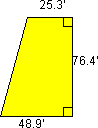+ Text Only Site
+ Non-Flash Version
+ Contact GlennBeginner's Guide to Aerodynamics
Drag Equation - Level 1

1. Click on The Drag Equation to open the appropriate slide. Study the equation and read the explanation of this concept. Then use the information to complete the questions below.

2. In the following table, identify each variable in the equation. State the appropriate units for each value:

#### English Units

D
Drag
Newtons
Pounds
Cd
Drag Coefficient
No units
No units
r
Density of air
kg/m3
slug/ft3
V
Velocity
m/s
ft\s
A
Reference Area
m2
ft2

A. What possible reference areas (A) can be used to compute the drag?
Total surface area of aircraft, frontal area of aircraft, or wing area.

B. If we want to compute the L/D (lift to drag ratio), which reference area would we want to use? Why?
Wing area, since lift is computed using the wing area.

C. Besides reference area, air density, and velocity, drag depends on several other values. What variable is used to model many of the complex dependencies of drag? What are some of the dependencies of drag incorporated in this value?
Cd - drag coefficient. Some of the dependencies of drag incorporated in this value are shape, inclination and some flow conditions.

3. Calculate the following problems which involve the drag of an aircraft: (Before computing, make sure all units agree)

A. Suppose you are flying an aircraft with the following wing shape and dimensions. What is the total wing area?A = 1/2h(b1 + b2) = 1/2 x 76.48(25.3 + 48.9) = 2834.44 ft2 for one wing
= 5668.88 ft2 for both wings

B. The thrust of the aircraft is 120,000 pounds and the air density is .00048 slugs/cu.ft. The current cruising speed is 450 ft/sec and we need to determine the drag coefficient of the aircraft. Find the Cd. (Hint: you may want to convert the original equation first)
Cd = 2D/(r x V2 x A) = 2(120,000)/(.00048)(450)2(5668.88) = .436

C. Weather conditions force the aircraft to descend to a level where the air density is .00076 slugs/cu.ft. The engine thrust increases to 250,000 pounds. At the new cruising altitude, what is the speed of the aircraft? (Hint: once again, you may want to convert the equation first)
V =sqrt(2D/Cd x r x A)=sqrt(2x(250,000)/(.436 x .00076 x 5668.88)) = 516 ft/sec

Related Pages:
Standards
Activity
Worksheet
Aerodynamics Activity Index
Aerodynamics Index+ Inspector General Hotline + Equal Employment Opportunity Data Posted Pursuant to the No Fear Act + Budgets, Strategic Plans and Accountability Reports + Freedom of Information Act + The President's Management Agenda + NASA Privacy Statement, Disclaimer, and Accessibility CertificationEditor: Tom Benson NASA Official: Tom Benson Last Updated: Thu, May 13 02:38:39 PM EDT 2021 + Contact Glenn